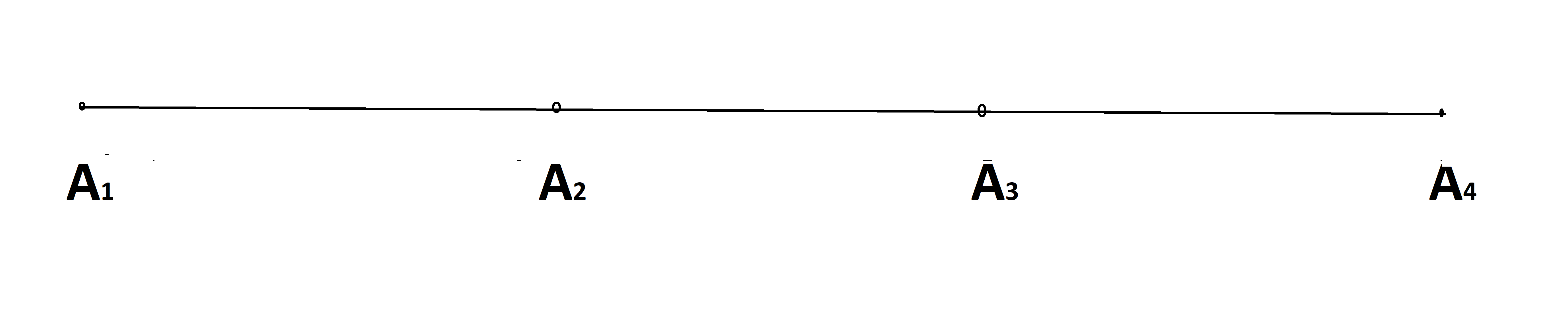# Combination of lines

There are $4$ co-linear pints $A_1,A_2,A_3$ and $A_4$Now suppose it take a set of line segments $B=\{\overline{A_1A_2},\overline{A_2A_3},\overline{A_3A_4}\}$ and a set of lines $C=\{\overline{A_1A_2},\overline{A_2A_4}\}$

What special about these sets is that no two line segments (in each of these sets) have more then one point in common or you can say that no two line segments have another segment overlapping on each other (for example line segment $\overline{A_2A_4}$ and $\overline{A_1A_3}$ are overlapping on each other on line segment $\overline{A_2A_3}$), let these line segments be called dis-joining lines

So now lets define something :

If you are given $n$ points $A_1,A_2,A_3...,A_n$ all of which are co-linear and you have a set of line segments $B$, now if all pair of line segments that are in $B$ are dis-joining and all lines, if summed together, forms line segment $\overline{A_1A_n}$ (For example in set $C$ in above example $\overline{A_1A_2}+\overline{A_2A_4}=\overline{A_1A_4}$) then set $B$ is called a Good set, else it will be called a Bad set.

How many distinct Good sets sets are possible if $n$ co-linear points are given? (Give a general formula)Note by Zakir Husain
11 months, 1 week ago

This discussion board is a place to discuss our Daily Challenges and the math and science related to those challenges. Explanations are more than just a solution — they should explain the steps and thinking strategies that you used to obtain the solution. Comments should further the discussion of math and science.

When posting on Brilliant:

• Use the emojis to react to an explanation, whether you're congratulating a job well done , or just really confused .
• Ask specific questions about the challenge or the steps in somebody's explanation. Well-posed questions can add a lot to the discussion, but posting "I don't understand!" doesn't help anyone.
• Try to contribute something new to the discussion, whether it is an extension, generalization or other idea related to the challenge.
• Stay on topic — we're all here to learn more about math and science, not to hear about your favorite get-rich-quick scheme or current world events.

MarkdownAppears as
*italics* or _italics_ italics
**bold** or __bold__ bold
- bulleted- list
• bulleted
• list
1. numbered2. list
1. numbered
2. list
Note: you must add a full line of space before and after lists for them to show up correctly
paragraph 1paragraph 2

paragraph 1

paragraph 2

[example link](https://brilliant.org)example link
> This is a quote
This is a quote
    # I indented these lines
# 4 spaces, and now they show
# up as a code block.

print "hello world"
# I indented these lines
# 4 spaces, and now they show
# up as a code block.

print "hello world"
MathAppears as
Remember to wrap math in $$ ... $$ or $ ... $ to ensure proper formatting.
2 \times 3 $2 \times 3$
2^{34} $2^{34}$
a_{i-1} $a_{i-1}$
\frac{2}{3} $\frac{2}{3}$
\sqrt{2} $\sqrt{2}$
\sum_{i=1}^3 $\sum_{i=1}^3$
\sin \theta $\sin \theta$
\boxed{123} $\boxed{123}$

## Comments

Sort by:

Top Newest

One $n$ -sized line-1 set.
Two separate $x,x-n$-sized lines-$x=1/2/3/...n-1$, n-1 sets.
Three: $x,y,n-x-y$, where there are $1+2+3+...+n-2$ possibilities for $(x,y)$, $\dfrac{(n-2)(n-1)}{2}$ sets.
...
Sum them up for the answer.

- 11 months, 1 week ago

Log in to reply

Umm...
$n+\sum _{i=1}^{n} \dfrac{\prod _{k=n} ^{i} (i-k)}{n}$

- 11 months ago

Log in to reply

$n+ \sum_{i=1}^{n}\dfrac{∏_{k=n}^{i}(i-k)}{n}=n+ \sum_{i=1}^{n}\dfrac{(i-n)...\red{(i-i)}}{n}=n+ \sum_{i=1}^{n}\dfrac{0}{n}=n+0=n$

- 11 months ago

Log in to reply

$n+\sum _{i=1}^{n} \dfrac{\prod _{k=1} ^{i} (n-k)}{i+1}$

- 11 months ago

Log in to reply

×

Problem Loading...

Note Loading...

Set Loading...